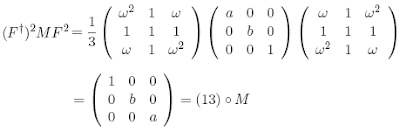On any diagonal matrix of (square root) mass eigenvalues, one instance of the squared Fourier transform acts as a simple permutation,and so clearly the fourth power of the transform is the identity. In other words, the discrete Fourier transform is like a square root of a basic 2-cycle or Pauli operator $\sigma_{X}$. The other choices for $F$ involve braiding elements.
What would a square root of a braid crossing look like? Geometrically, considering the element of $B_{2}$ as a map between bars with two points, the square root is, instead of a rotation of $\pi$ for the bar, a rotation of $\pi/2$. This configuration lines up the points on the bottom bar so that the strands appear to come together in a diagram that usually represents Hopf algebraic multiplication in a category, only now the points are still separated in the third dimension.